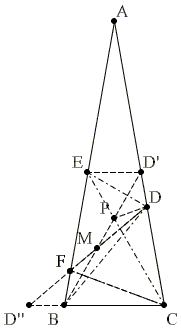# The 80-80-20 Triangle Problem, Solution #2Let ABC be an isosceles triangle (AB = AC) with ∠BAC = 20°. Point D is on side AC such that ∠CBD = 50°. Point E is on side AB such that ∠BCE = 60°. Find the measure of ∠CED.

Solution

The proof exploits the ideas used by Mariano Perez de la Cruz in solving a related problem.Draw CF, F on AB, with ∠BCF = 20°. Then ΔBFC is isosceles: BC = CF.

ΔBCD is also isosceles because

∠BDC = 180° - 80° - 50° = 50° = ∠CBD

Hence, BC = CD and so CD = CF.

It follows that ΔCDF is isosceles with the apex ∠DCF = 60°. Hence, ΔCDF is in fact equilateral.

Let ∠CBD' = 60°, with D' on AC. P the intersection of BD' and CE. ΔCPB is equilateral and maps by a rotation around C onto ΔCDF. Therefore, if M is the intersection of DF and BP, CM is the bisector of ∠ACB.

Now, consider a reflection in CM: D maps onto B, P onto F; let D' map on D'', D'' is on the extension of BC. Angle CFD'' (= ∠CPD') = 120°, ∠FCD'' = 20°. Thus ∠CD''F = 40°. Angle FBD'' = 100° making ∠BFD'' = 40° and Δ BFD'' isosceles: BF = BD''. Importantly, DP = DD'.

Since ΔEPD' is equilateral, the quadrilateral EPDD' is a kite, its diagonals are perpendicular. It follows that ED is the bisector of angles PED' and PDD'. In particular, ∠PED = 30°.

In other words, ∠CED = 30°.### The 80-80-20 Triangle Problem